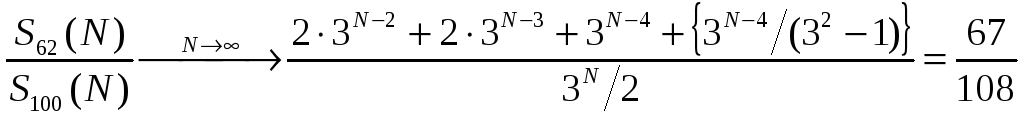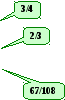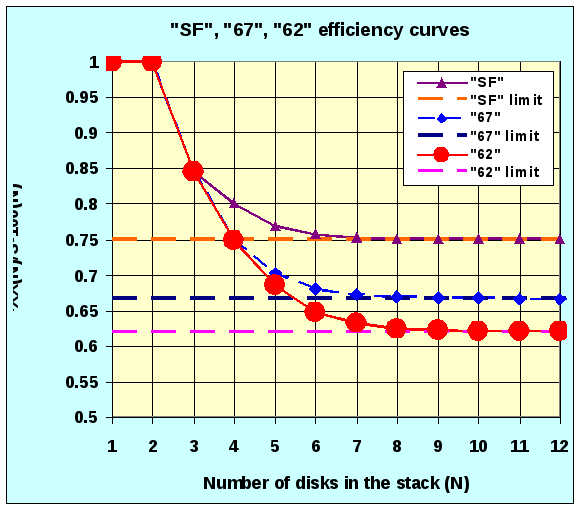# The Magnetic Tower of Hanoi

 Table 9: Number of disk-moves for the "62" Algorith solving the Magnetic Tower of Hanoi puzzle (Figure 11, Equation 18 and Equation 19). Table 9 lists the number of moves of the k-th disk for the first eight stack "heights". Looking at the number of moves for the "62" Algorithm as listed in Table 9, and comparing the numbers to the numbers listed in Table 6 for the "67" Algorithm, we indeed see some additional savings. For example, the total number of moves to solve the 8-disk MToH puzzle using the "67" Algorithm is 2194 while using the "62" Algorithm the number is only 2050. The "100" Algorithm, by the way, (the algorithm that solves a Colored-MToH), calls for 3280 moves (Table 4). For the limit of the "duration-ratio" of "62" vs. "100", we retain the high N-powers of 3 to find –(20)Download 2.78 Mb.Share with your friends: